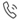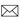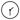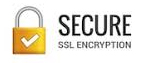SUBJECTS# Books on Mathematics

Total 2345 Books Found
 ( Showing Book 1 to 80 ) Next
10%
 Measures of Information and Their Applications by Kapur, J.N. Rs 550.00 Rs 495.00
10%
 Theory and Problems of Combinatorics by Vasudev, C. Rs 299.00 Rs 269.10
10%
 The Meaning of Relativity by Einstein, Albert Rs 95.00 Rs 85.50
10%
 Engineering Mathematics, Volume-I by Rao, H.S.G. Rs 295.00 Rs 265.50
10%
 Finite Element Methods vs. Classical Methods by Rao, H.S.G. Rs 195.00 Rs 175.50
10%
 Engineering Mathematics-I (as per RTU & Other Universities) by Gupta, C.B. Rs 175.00 Rs 157.50
10%
 Mathematics-III (B.Tech., III Semester (EE & ECE Branch) Students of RTU and Other Universities by Gupta, C.B. Rs 195.00 Rs 175.50
10%
 Mathematical Physics for Engineers by Bera, R.K. Rs 795.00 Rs 715.50
10%
 A Guide to MATLAB Object-Oriented Programming by Register, Andy H. Rs 395.00 Rs 355.50
10%
 GATE Mathematics ( For, CS, IT & MCA Students ) by Jana, Dipak Kumar Rs 195.00 Rs 175.50
10%
 Engineering Mathematics-II (RTU) by Gupta, C.B. Rs 199.00 Rs 179.10
10%
 University Algebra Through 600 Solved Problems by Gopalakrishnan, N. S. Rs 175.00 Rs 157.50
10%
 A Textbook of Engineering Mathematics-I (UPTU) by Gangwar, H.S. Rs 275.00 Rs 247.50
10%
 A Textbook of Discrete Mathematics (WBUT) by Samanta, Guruprasad Rs 199.00 Rs 179.10
10%
 First Course in Linear Algebra by Bhattacharya, P.B. Rs 299.00 Rs 269.10
10%
 Inferential Problems in Survey Sampling by Mukhopadhyay, Parimal Rs 799.00 Rs 719.10
10%
 A Textbook of Engineering Mathematics-II  by Samanta, Guruprasad Rs 275.00 Rs 247.50
10%
 Computational Methods for Partial Differential Equations by Jain, M.K. Rs 275.00 Rs 247.50
10%
 Engineering Mathematics-II (VTU) by Ganesh, A. Rs 195.00 Rs 175.50
10%
 The Rotation and Lorentz Groups and Their Representations for Physicists by Rao, K.N. Srinivasa Rs 799.00 Rs 719.10
10%
 Matrix Methods of Structural Analysis by Kanchi, M.B. Rs 350.00 Rs 315.00
10%
 A Textbook of Engineering Mathematics- III (MAKAUT)  by Samanta, Guruprasad  Rs 399.00 Rs 359.10
10%
 A Problem Book in Algebra by Krechmar, V.A. Rs 199.00 Rs 179.10
10%
 An Elementary Treatise on Pure Geometry by Russell, J.W. Rs 199.00 Rs 179.10
10%
 JEE Main Plus! Mathematics by Saxena, Nikhil Rs 550.00 Rs 495.00
10%
 Lebesgue Measure and Integration by Jain, P.K. Rs 699.00 Rs 629.10
10%
 Engineering Statistics and Linear Algebra by Mamatha, A S Rs 375.00 Rs 337.50
10%
 University Algebra by Gopalakrishnan, N.S. Rs 299.00 Rs 269.10
10%
 Programmed Statistics  (Questions-Answers) by Agarwal, B.L. Rs 499.00 Rs 449.10
10%
 Principles of Real Analysis by Malik, S.C. Rs 399.00 Rs 359.10
10%
 Multivariate Calculus Through Linear Algebra by Joshi, K.D. Rs 599.00 Rs 539.10
10%
 Higher Algebra by Barnard, S. Rs 399.00 Rs 359.10
10%
 Elements of Geometry by Barnard, S. Rs 299.00 Rs 269.10
10%
 Relativity : The Special and General Theory by Einstein, Albert Rs 99.00 Rs 89.10
10%
 Hyperbolic Functions by Becker, George F. Rs 199.00 Rs 179.10
10%
 The Elements of Statics and Dynamics Part-II (Dynamics) by Loney, S.L. Rs 150.00 Rs 135.00
10%
 An Introduction to the Geometry of N Dimensions by Sommerville, D.M.Y. Rs 99.00 Rs 89.10
10%
 First Year Algebra by Wells, Webster Rs 150.00 Rs 135.00
10%
 Elementary Calculus by Woods, Frederick S. Rs 199.00 Rs 179.10
10%
 A Textbook of Computer Based Numerical and Statistical Techniques (As per UPTU Syllabus) by Jaiswal, A.K. Rs 199.00 Rs 179.10
10%
 Metric Space & Complex Analysis by Banerjee, A.K. Rs 499.00 Rs 449.10
10%
 Operations Research for Management by Shenoy, G.V. Rs 350.00 Rs 315.00
10%
 Matrices and Tensors in Physics by Joshi, A.W. Rs 375.00 Rs 337.50
10%
 Elements of Group Theory for Physicists by Joshi, A.W. Rs 399.00 Rs 359.10
10%
 Computer Graphics in Mathematical Approaches by Kothari, D P Rs 399.00 Rs 359.10
10%
 Functional Analysis by Limaye, Balmohan. V. Rs 550.00 Rs 495.00
10%
 IIT-JEE Unsolved Papers: Physics, Chemistry, Mathematics by New Age Editorial Board Rs 225.00 Rs 202.50
10%
 Selected Statistical Tests by Rajagopalan, V Rs 699.00 Rs 629.10
10%
 A Textbook of Analytical Geometry of Two Dimensions by Jain, P K Rs 299.00 Rs 269.10
10%
 A Textbook of Analytical Geometry of Three Dimensions by Jain, P K Rs 299.00 Rs 269.10
10%
 Numerical Solutions of Differential Equations by Jain, M.K. Rs 550.00 Rs 495.00
10%
 Numerical Analysis by Rao, Shanker G. Rs 450.00 Rs 405.00
10%
 Discrete Mathematics Structures by Rao, Shanker G. Rs 399.00 Rs 359.10
10%
 Design and Analysis of Experiments by Das, M.N. Rs 1299.00 Rs 1169.10
10%
 Research Methodology: Methods and Techniques by Kothari, C.R. Rs 399.00 Rs 359.10
10%
 Linear Estimation and Design of Experiments by Joshi, D.D. Rs 875.00 Rs 787.50
10%
 Vectors and Geometry by Pandey, G.S. Rs 275.00 Rs 247.50
10%
 Vector Analysis with Applications by Ashraf Ali, MD. Rs 375.00 Rs 337.50
10%
 General Measure and Integration by Jain, P.K. Rs 599.00 Rs 539.10
10%
 Theory of Bases in Banach Spaces by Jain, P.K. Rs 699.00 Rs 629.10
10%
 General Topology by Murdeshwar, M. G. Rs 899.00 Rs 809.10
10%
 Probability Distributions of Two Random Variables by Uppal, S.M. Rs 475.00 Rs 427.50
10%
 Stochastic Models: Analysis and Applications by Bhat, B.R. Rs 999.00 Rs 899.10
10%
 Modern Probability Theory by Bhat, B.R. Rs 350.00 Rs 315.00
10%
 Hydraulic Machines by Kothari, D.P. Rs 999.00 Rs 899.10
10%
 Theory and Analysis of Sample Survey Design by Singh, Daroga Rs 399.00 Rs 359.10
10%
 Functional Analysis by Jain, P.K. Rs 399.00 Rs 359.10
10%
 Optimization Methods in Operations Research and Systems Analysis by Mital, K.V. Rs 399.00 Rs 359.10
10%
 Statistical Analysis of Non-Normal Data by Deshpande, J.V. Rs 999.00 Rs 899.10
10%
 Handbook of Mathematical Formulae and Scientific Data For Engineers by Kothandaraman, C.P. Rs 199.00 Rs 179.10
10%
 The Elements of Complex Analysis by Choudhary, B. Rs 299.00 Rs 269.10
10%
 Applied Numerical Methods Using MATLAB by Dukkipati, Rao V. Rs 799.00 Rs 719.10
10%
 Numerical Methods: Problems and Solutions by Jain, M.K. Rs 475.00 Rs 427.50
10%
 Numerical Methods by Dukkipati, Rao V. Rs 399.00 Rs 359.10
10%
 Stochastic Processes by Medhi, J. Rs 499.00 Rs 449.10
10%
 Basic Statistics by Agarwal, B.L. Rs 599.00 Rs 539.10
10%
 Optimization using Linear Programming by Meitei, A.J. Rs 395.00 Rs 355.50
10%
 Challenge and Thrill of Pre-College Mathematics by Krishnamurthy, V Rs 499.00 Rs 449.10
10%
 Numerical Methods for Scientific and Engineering Computation by Jain, M.K. Rs 699.00 Rs 629.10
10%
 Shodh Paddhati by Kothari, C.R. Rs 395.00 Rs 355.50SupportPhone : +91-9266663909Email : support [at] bestbookmart.comTiming : 10:00 AM to 6:00 PM (Mon-Fri)